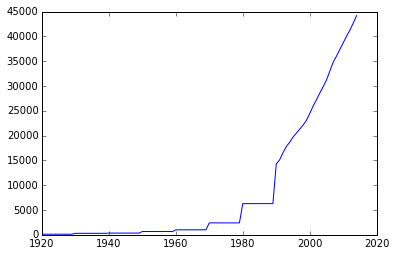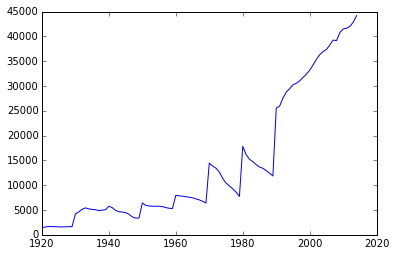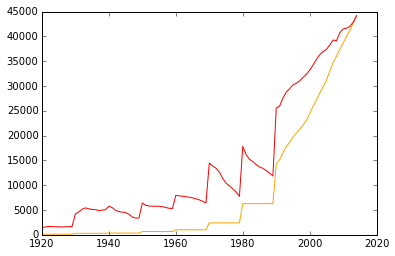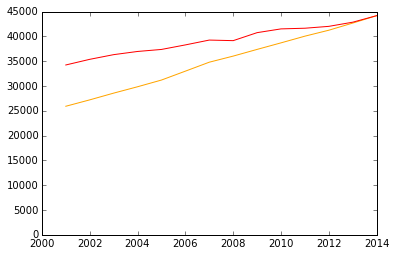# Stanford tuition fun¶

"The Second Tuition Bomb" - The Stanford Illustrated Review (books.google.com)

Stanford lists the historical tuition prices here: Finances facts

Let's see how charting can better illustrate the data.

## Download the data¶

In :
# scraping stanford's tuition page

import pandas as pd
import csv
from lxml import html
import requests
import re
# live site is at: "http://facts.stanford.edu/administration/finances"
url = 'http://stash.compjour.org/mirrors/facts.stanford.edu/administration/finances.html'
resp = requests.get(url)
doc = html.fromstring(resp.text)
table = doc.cssselect('table')

rows = []
for trs in table.cssselect('tr')[1:]:
yr, cost = [t.text for t in trs]
# cut off the "1959" part of "1950-1959"
rows.append( [int(yr.split('-')), int(re.sub('\D', '', cost))])

# alternatively
# rows = [( int(tds.text.split('-')), int(re.sub('\D', '', tds.text))) for tds in
#              [trs for trs in table.cssselect('tr')[1:]]]


Now we need to fill in the gaps between the decades; for years in which no tuition is specified, we assume it's the same tuition as the previous year.

Warning: convoluted code to follow

In :
# make a row for every year
tuition_rows = []
for row in rows:
if len(tuition_rows) > 0:
lastyr, lastcost = tuition_rows[-1]
tuition_rows.extend([[lastyr + i, lastcost] for i in range(1, row - lastyr)])
tuition_rows.append(row)

# Now make a dataframe
tuition_df = pd.DataFrame(tuition_rows, columns = ['year', 'tuition'])
tuition_df.head()

Out:
year tuition
0 1920 120
1 1921 120
2 1922 120
3 1923 120
4 1924 120

### Make an inflation-calcuation funciton¶

Download some CPI/inflation data from OKFN

US Consumer Price Index and Inflation (CPI)

In :
########################
# Set up inflation calculator

url = 'https://raw.githubusercontent.com/datasets/cpi-us/master/data/cpiai.csv'
cpidata = list(csv.reader(requests.get(url).text.splitlines()))
cpidf = pd.DataFrame(cpidata[1:], columns = cpidata)
cpidf = pd.DataFrame.convert_objects(cpidf, convert_dates = 'coerce', convert_numeric = True)
cpimean_df = cpidf.groupby(cpidf['Date'].map(lambda x: x.year)).mean()

def adjust_for_inflation(amt, from_year, to_year):
ratio = cpimean_df['Index'][to_year] / cpimean_df['Index'][from_year]
return round(ratio * amt, 2)

tuition_df['adjusted_tuition'] = tuition_df.apply(lambda x: adjust_for_inflation(x['tuition'], x['year'], 2014), axis=1)
tuition_df.head(15)

/Users/dtown/anaconda/lib/python3.4/site-packages/pandas/core/index.py:667: FutureWarning: scalar indexers for index type Int64Index should be integers and not floating point
type(self).__name__),FutureWarning)

Out:
year tuition adjusted_tuition
0 1920 120 1400.58
1 1921 120 1572.54
2 1922 120 1675.82
3 1923 120 1646.33
4 1924 120 1639.12
5 1925 120 1600.19
6 1926 120 1585.87
7 1927 120 1617.09
8 1928 120 1635.94
9 1929 120 1635.94
10 1930 300 4202.08
11 1931 300 4614.23
12 1932 300 5144.15
13 1933 300 5425.89
14 1934 300 5243.45

Now we can chart.

In :
import matplotlib.pyplot as pyplot
# this part is needed if you are doing this in an iPython notebook
%matplotlib inline


Sans inflation:

In :
pyplot.plot(tuition_df['year'], tuition_df['tuition'])

Out:
[<matplotlib.lines.Line2D at 0x10d456208>]Now with adjustments for inflation:

In :
pyplot.plot(tuition_df['year'], tuition_df['adjusted_tuition'])

Out:
[<matplotlib.lines.Line2D at 0x10d620cc0>]On the same chart:

In :
pyplot.plot(tuition_df['year'], tuition_df['tuition'], label = 'Unadjusted', color = 'orange')
pyplot.plot(tuition_df['year'], tuition_df['adjusted_tuition'], label = 'Adjusted', color = 'red')

Out:
[<matplotlib.lines.Line2D at 0x10d5991d0>]Truncated:

In :
xdf = tuition_df[tuition_df['year'] > 2000]

pyplot.plot(xdf['year'], xdf['tuition'], label = 'Unadjusted', color = 'orange')
pyplot.plot(xdf['year'], xdf['adjusted_tuition'], label = 'Adjusted', color = 'red')
pyplot.ylim(ymin = 0)

Out:
(0, 45000.0)In [ ]: